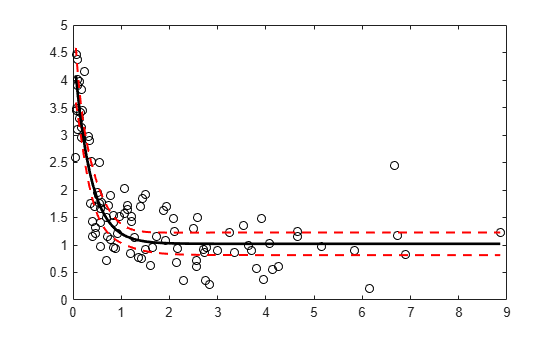# predict

Class: NonLinearModel

Predict response of nonlinear regression model

## Syntax

```ypred = predict(mdl,Xnew) [ypred,yci] = predict(mdl,Xnew) [ypred,yci] = predict(mdl,Xnew,Name,Value) ```

## Description

`ypred = predict(mdl,Xnew)` returns the predicted response of the `mdl` nonlinear regression model to the points in `Xnew`.

```[ypred,yci] = predict(mdl,Xnew)``` returns confidence intervals for the true mean responses.

```[ypred,yci] = predict(mdl,Xnew,Name,Value)``` predicts responses with additional options specified by one or more `Name,Value` pair arguments.

## Input Arguments

 `mdl` Nonlinear regression model, constructed by `fitnlm`. `Xnew` Points at which `mdl` predicts responses. If `Xnew` is a table or dataset array, it must contain the predictor names in `mdl`.If `Xnew` is a numeric matrix, it must have the same number of variables (columns) as was used to create `mdl`. Furthermore, all variables used in creating `mdl` must be numeric.

### Name-Value Arguments

Specify optional pairs of arguments as `Name1=Value1,...,NameN=ValueN`, where `Name` is the argument name and `Value` is the corresponding value. Name-value arguments must appear after other arguments, but the order of the pairs does not matter.

Before R2021a, use commas to separate each name and value, and enclose `Name` in quotes.

 `Alpha` Positive scalar from `0` to `1`. Confidence level of `yci` is 100(1 – `alpha`)%. Default: `0.05`, meaning a 95% confidence interval. `Prediction` Type of prediction: `'curve'` — `predict` predicts confidence bounds for the fitted mean values.`'observation'` — `predict` predicts confidence bounds for the new observations. This results in wider bounds because the error in a new observation is equal to the error in the estimated mean value, plus the variability in the observation from the true mean. For details, see `polyconf`. Default: `'curve'` `Simultaneous` Logical value specifying whether the confidence bounds are for all predictor values simultaneously (`true`), or hold for each individual predictor value (`false`). Simultaneous bounds are wider than separate bounds, because it is more stringent to require that the entire curve be within the bounds than to require that the curve at a single predictor value be within the bounds. For details, see `polyconf`. Default: `false` `Weights` Vector of real, positive value weights or a function handle. If you specify a vector, then it must have the same number of elements as the number of observations (or rows) in `Xnew`.If you specify a function handle, then the function must accept a vector of predicted response values as input, and return a vector of real positive weights as output. Given weights, `W`, `predict` estimates the error variance at observation `i` by `MSE*(1/W(i))`, where MSE is the mean squared error. Default: No weights

## Output Arguments

 `ypred` Predicted mean values at `Xnew`. `ypred` is the same size as each component of `Xnew`. `yci` Confidence intervals, a two-column matrix with each row providing one interval. The meaning of the confidence interval depends on the settings of the name-value pairs.

## Examples

expand all

Create a nonlinear model of car mileage as a function of weight, and predict the response.

Create an exponential model of car mileage as a function of weight from the `carsmall` data. Scale the weight by a factor of 1000 so all the variables are roughly equal in size.

```load carsmall X = Weight; y = MPG; modelfun = 'y ~ b1 + b2*exp(-b3*x/1000)'; beta0 = [1 1 1]; mdl = fitnlm(X,y,modelfun,beta0);```

Create predicted responses to the data.

```Xnew = X; ypred = predict(mdl,Xnew);```

Plot the original responses and the predicted responses to see how they differ.

```plot(X,y,'o',X,ypred,'x') legend('Data','Predicted')```Create a nonlinear model of car mileage as a function of weight, and examine confidence intervals of some responses.

Create an exponential model of car mileage as a function of weight from the `carsmall` data. Scale the weight by a factor of 1000 so all the variables are roughly equal in size.

```load carsmall X = Weight; y = MPG; modelfun = 'y ~ b1 + b2*exp(-b3*x/1000)'; beta0 = [1 1 1]; mdl = fitnlm(X,y,modelfun,beta0);```

Create predicted responses to the smallest, mean, and largest data points.

```Xnew = [min(X);mean(X);max(X)]; [ypred,yci] = predict(mdl,Xnew)```
```ypred = 3×1 34.9469 22.6868 10.0617 ```
```yci = 3×2 32.5212 37.3726 21.4061 23.9674 7.0148 13.1086 ```

Generate sample data from the nonlinear regression model

`$y={b}_{1}+{b}_{2}\mathrm{exp}\left(-{b}_{3}x\right)+ϵ$`

where ${b}_{1}$, ${b}_{2}$, and ${b}_{3}$ are coefficients, and the error term $ϵ$ is normally distributed with mean 0 and standard deviation 0.5.

```modelfun = @(b,x)(b(1)+b(2)*exp(-b(3)*x)); rng('default') % For reproducibility b = [1;3;2]; x = exprnd(2,100,1); y = modelfun(b,x) + normrnd(0,0.5,100,1);```

Fit the nonlinear model using robust fitting options.

```opts = statset('nlinfit'); opts.RobustWgtFun = 'bisquare'; b0 = [2;2;2]; mdl = fitnlm(x,y,modelfun,b0,'Options',opts);```

Plot the fitted regression model and simultaneous 95% confidence bounds.

```xrange = [min(x):.01:max(x)]'; [ypred,yci] = predict(mdl,xrange,'Simultaneous',true); figure() plot(x,y,'ko') % observed data hold on plot(xrange,ypred,'k','LineWidth',2) plot(xrange,yci','r--','LineWidth',1.5)``````S = load('reaction'); X = S.reactants; y = S.rate; beta0 = S.beta;```

Specify a function handle for observation weights, then fit the Hougen-Watson model to the rate data using the specified observation weights function.

```a = 1; b = 1; weights = @(yhat) 1./((a + b*abs(yhat)).^2); mdl = fitnlm(X,y,@hougen,beta0,'Weights',weights);```

Compute the 95% prediction interval for a new observation with reactant levels [100,100,100] using the observation weight function.

```[ypred,yci] = predict(mdl,[100,100,100],'Prediction','observation', ... 'Weights',weights)```
```ypred = 1.8149 ```
```yci = 1×2 1.5264 2.1033 ```

## Tips

 Lane, T. P. and W. H. DuMouchel. “Simultaneous Confidence Intervals in Multiple Regression.” The American Statistician. Vol. 48, No. 4, 1994, pp. 315–321.

 Seber, G. A. F., and C. J. Wild. Nonlinear Regression. Hoboken, NJ: Wiley-Interscience, 2003.